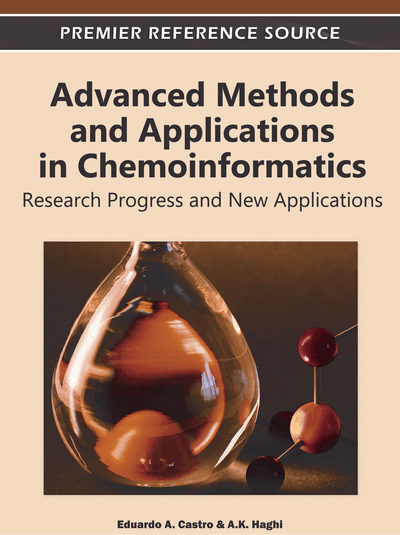# A QSAR/QSPR Study with Graph-Theoretical Indices based on a New Type of Vertex Degree

Lionello Pogliani (Università della Calabria, Italy)
DOI: 10.4018/978-1-60960-860-6.ch006
Available
\$37.50
No Current Special Offers

## Abstract

Valence molecular connectivity indices are indices based on the concept of valence delta, d v, that can be derived from general chemical graphs or chemical pseudographs. A general graph or pseudograph is a graph with multiple edges and loops and it can be used to encode, through the valence delta, chemical entities like the sigma-, pi- and non-bonding n-electrons. Two other graph-theoretical concepts that can also be derived from chemical pseudographs are the intrinsic (I) and the electrotopological state (E) values that are the main tools used to define the valence delta of the pseudoconnectivity indices, ?I,S. Complete graphs can, instead, be used to encode, through a new type of valence delta, the core electrons of any type of atoms in a molecule. The connectivity indices either valence connectivity or pseudoconnectivity indices are the starting point to develop, by the aid of a dual procedure, the dual connectivity indices, i.e., the dual connectivity, valence connectivity and pseudoconnectivity indices. The dual indices show the interesting property that not only some of them can assume negative values but also that they can cover a wide range of numerical values. Graph concepts can also be used to deal with the problem of the hydrogen contribution in hydrogen depleted chemical graphs, which are the normal type of graphs used in chemistry. For this purpose a perturbation parameter can be introduced into the definition of the valence delta that allows to differentiates among compounds with similar hydrogen-suppressed chemical graphs but different number of hydrogen atoms, like CH3F and BH2F. The new definition of the central parameter of the molecular connectivity theory, the valence delta, consent to define of a completely new set of connectivity indices, which can be distinguished by their configuration and that can advantageously be used to model different properties and activities of compounds.
Chapter Preview
Top

## Introduction

The many new types of molecular connectivity indices (Pogliani, 2000, 2002, 2004, 2005, 2006, 2007, 2009, 2010; Garcia-Domenech et al. 2008), which will be discussed and used for model purposes in this paper are defined by a family of formally similar algorithms, first formulated by Randić (Randić, 1975) and then developed into a general theory, known as the molecular connectivity (MC) theory, by Kier and Hall (Kier & Hall, 1986, 1999). This theory has, since then, been enriched and discussed in many ways (Pogliani, 2000; Garcia-Domenech, et al., 2008; Trinajstić, 1992; Devillers & Balaban, 1999, Todeschini & Consonni, 2000; Diudea, 2000; Estrada, 2001; Li & Gutman, 2006). The central parameter of the molecular connectivity theory is the valence delta number, δv, which, in the hands of Pogliani, has undergone a major transformation (Pogliani, 2000-2010; Garcia-Domenech, et al. 2008). This graph-theoretical number, shown in eq. (1) actually encodes not only the sigma-, pi- and non-bonding n-electrons but also the core electrons and the contribution of the suppressed hydrogen atoms,

(1)

Parameter δv(ps) is the vertex degree number of an atom in a pseudograph. Parameter q equals 1 or p; parameter p⋅r equals the sum of all vertex degrees in a complete graph, and it equals twice the number of its connections. Notice that parameter q has fixed values and it is not used as an optimizing parameter, something like Randić’s variable index (Randić & Basak, 2001). The fact that q = 1 or p has the consequence that four possible sets of molecular connectivity indices can be obtained and they are: for q = 1 and p = odd (1, 3, 5, ..) a Kp-(p-odd) set of indices is obtained, for q = 1 and p = sequential (1, 2, 3, …) a Kp-(p-seq) set is obtained, for q = p and p = odd a Kp-(pp-odd) set is obtained, and for q = p and p = seq a Kp-(pp-seq) set of indices is obtained. The rationale of this distinction resides in the fact that in many cases MC indices derived with p odd-valued show a superior model quality. Perturbation parameter fδ takes care of the suppressed hydrogen atoms in a chemical graph or pseudograph, and is defined in the following way

fδ = [δvm(ps)- δv(ps)]/δvm(ps) = 1 - δv(ps)/δvm(ps) = nHvm(ps) (2)

Here, δvm(ps) is the maximal δv(ps) value a heteroatom can have in a chemical HS-pseudograph when all bonded hydrogens are substituted by heteroatoms, and nH equals the number of hydrogen atoms normally bonded to any heteroatom (C inclusive).

## Complete Chapter List

Search this Book:
Reset